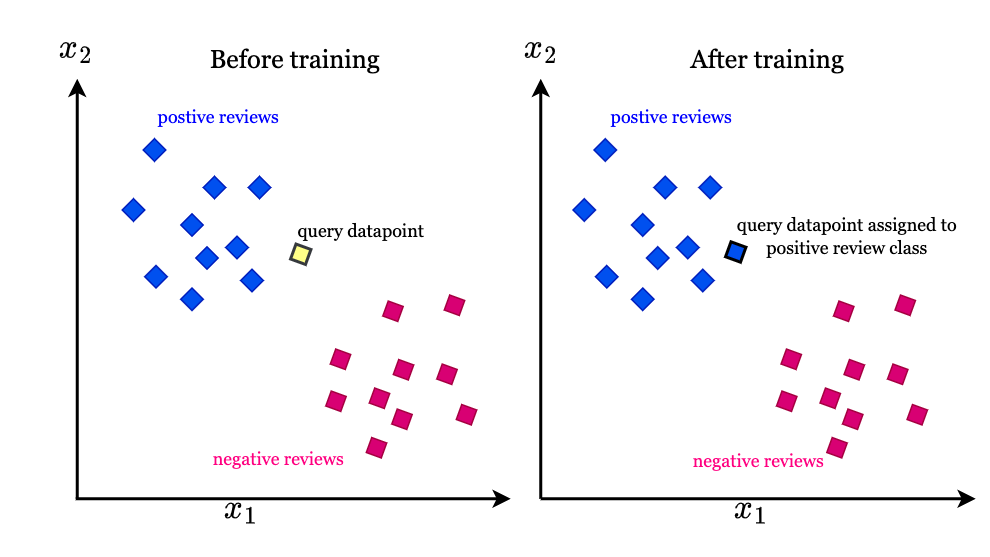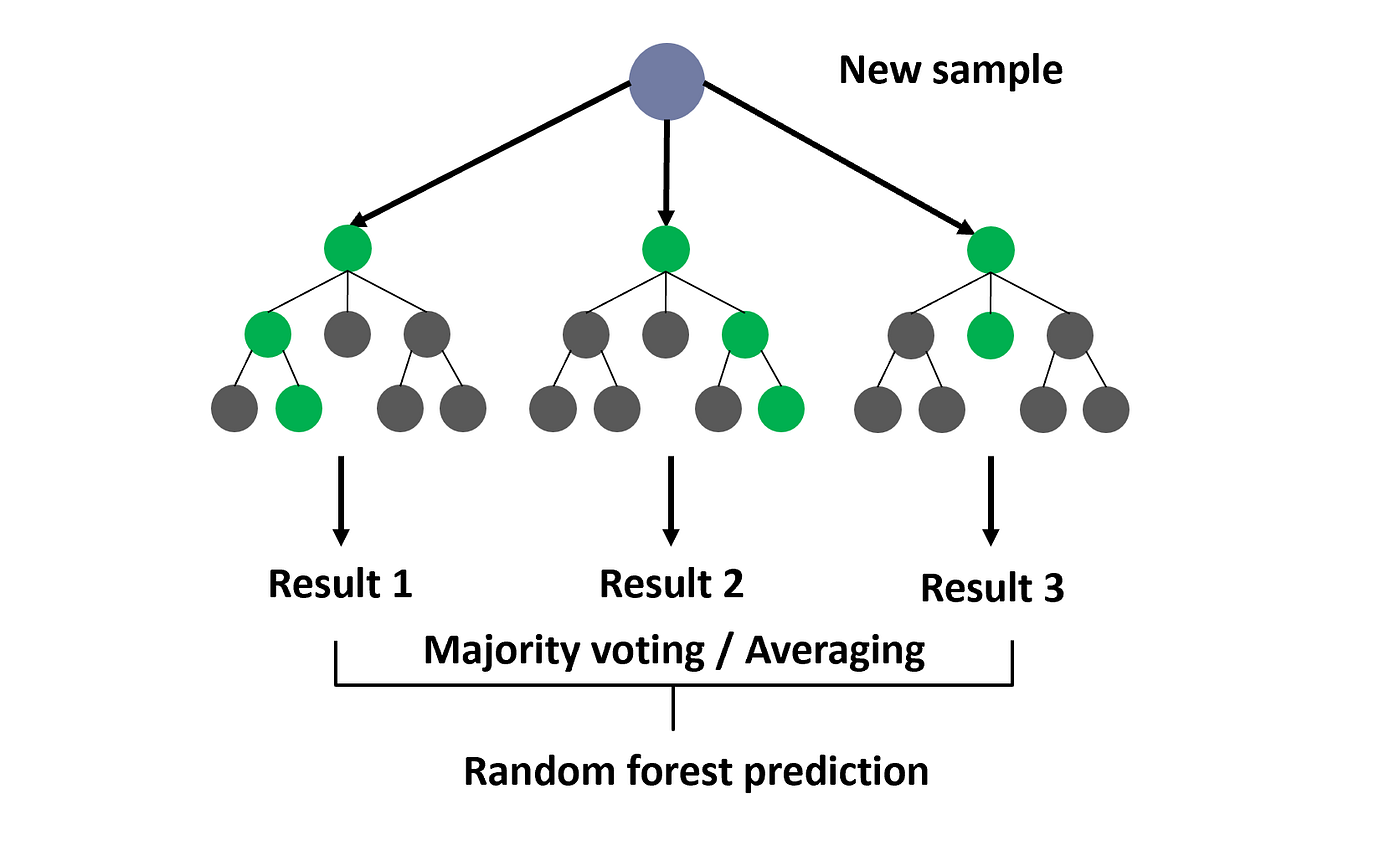0 39

# Data Mining and Warehousing - 4

What is Supervised Learning? Supervised learning is a machine learning task of inferring a function from labelled traini...

## What is Supervised Learning?

Supervised learning is a machine learning task of inferring a function from labelled training data.The training data consists of a set of training examples. Each example is a pair consisting of an input object and a desired output value also called the supervisory signal.

A supervised learning algorithm analyzes the training data and produces an inferred function, which can be used for mapping new examples. The inferred function should predict the correct output value for any valid input object.

The goal of supervised learning is to create a model that generalizes from the training data to unseen examples to produce reliable predictions.

### Main Steps in Supervised Learning

1. Acquire a dataset with labelled examples - The dataset consists of input objects along with the correct, known output values for those inputs.

2. Split the dataset into training and test sets - The training set is used to train the model. The test set is used to evaluate the model's performance.

3. Select a supervised learning algorithm - Common algorithms are decision trees, SVMs, neural networks, Bayesian classifiers etc.

4. Train the model using the training set - The algorithm learns from the labelled training examples to infer a function that maps inputs to outputs.

5. Evaluate the model using the test set - The model's accuracy and performance are measured on unseen data i.e. the test set.

6. Improve the model if needed - The model can be refined using techniques like parameter tuning, adding features, regularization etc.

7. Use the model to predict new, unseen examples.

## Classification Algorithms

Classification algorithms are machine learning models that are used to assign data points into predefined categories or classes. They take input data and map it to one of several output classes.

## Statistical-based Algorithms

Statistical-based classification algorithms use statistical techniques to model the relationship between features and classes. They make assumptions about the underlying data distribution and use probability and statistics to perform classification.

The main techniques used are:

### Bayesian Classification:

• It is based on Bayes' theorem and the assumption of conditional independence between features.

• It calculates the posterior probability P(c|x) for each class c given a data point x using Bayes' theorem: P(c|x) = P(x|c) * P(c) / P(x)

• P(x|c) is the likelihood, which is the probability of observing x given class c. It is estimated from the training data for each class.

• P(c) is the prior probability of class c, which can be calculated from the training data.

• P(x) is the probability of data point x. It is constant for a given data point.

• The data point is assigned to the class with the highest posterior probability P(c|x).

• Simple and easy to implement

• Works well for high dimensional data due to the independence assumption

• Requires small amounts of training data

• Performance degrades if the independence assumption is violated

• Sensitive to outliers in the training data

### Logistic Regression:

• It models the probability P(y=1|x) of a data point belonging to a class as a logistic function of the weighted linear combination of features.

• The weights are estimated from the training data using maximum likelihood estimation.

• A data point is classified as the class with the highest predicted probability.

• The predicted probability ranges from 0 to 1, making it suitable for classification.• Interpretable as it provides weights for each feature

• Works well even when features are correlated

• Scales well to a large number of features

• Assumes a linear relationship between features and output

• Requires large amounts of training data

## Distance-based algorithms

Distance-based classifiers assign unlabelled instances to the class of their nearest labelled neighbour (s) based on a distance metric. The two main distance-based classifiers are:

### K Nearest Neighbors (KNN)

• KNN is a simple yet effective classification technique.

• It stores all available cases and classifies new cases based on a similarity measure (distance functions).

• Given a test instance, KNN finds its K nearest neighbours in the training set. The test instance is then assigned to the class most common among those K neighbours.

• K is a positive integer, typically small.

• A larger K reduces the effect of noise on the classification but makes boundaries between classes less distinct.

• The optimal K depends on the data and is chosen using cross-validation.• Simple implementation and easy to understand.

• Works well when the decision boundary is nonlinear.

• Computationally expensive, especially for large training sets.

• Sensitive to the distance metric used.

• All training samples are stored, requiring more memory.

### Example-based classification

• Example-based classifiers use the entire training set as prototypes.

• They assign a test instance to the class of its nearest neighbour.

• This is equivalent to KNN with K = 1.

• It is a special case of KNN and shares its advantages and disadvantages.

## Decision tree-based algorithms

### Decision Trees

• Decision trees are a popular classification technique that mimics the decision-making process of humans.

• They have a tree-like structure, with internal nodes representing features, branches representing decisions and leaf nodes representing class labels.

• The decision tree is constructed in a top-down recursive divide-and-conquer manner.

• It starts with the root node containing all training samples and splits them into two or more homogeneous sets based on the most discriminative feature.

• This process repeats on each derived subsample until the maximum depth is reached or all samples belong to the same class.### Random Forests

• Random forests are an ensemble of decision trees trained on random subsets of the data.

• Each tree is constructed using a random subset of features.

• The final class is determined by taking the majority vote of all the trees.

• Random forests reduce the variance of decision trees and are less prone to overfitting.

• They achieve better accuracy and generalization capability compared to single decision trees.• Handle both numerical and categorical data.

• Requires little data preparation.

• Random forests are more accurate and robust.

• Interpretability is reduced due to the ensemble of trees.

• Performance degrades with high dimensional data.

• Computationally intensive for large datasets.

## Neural network-based algorithms

Neural networks are biologically inspired models that mimic the workings of the human brain. They are well suited for complex classification tasks.

### Multi-Layer Perceptrons (MLPs)

• MLPs are feedforward neural networks with one or more hidden layers between the input and output layers.

• They use a backpropagation algorithm for supervised training.

• MLPs can model complex non-linear decision boundaries to accurately classify data.

• They are universal function approximators and can model any function with enough neurons.### Radial Basis Function Networks (RBFNs)

• RBFNs use radial basis functions as activation functions of hidden layer neurons.

• The output layer uses a linear activation function.

• RBFNs can learn and generalize faster than MLPs during training.

• They tend to give good results for smaller training sets.• Can model complex non-linear decision boundaries.

• Universal function approximators.

• Require large training data to avoid overfitting.

• Prone to get stuck in local optima during training.

• Computationally expensive.

• Difficult to interpret the learned model.

## Rule-based algorithms

Rule-based classifiers generate rules from the training data and use them to classify new instances.

### Rule Induction

![PDF] Design and analysis of scalable rule induction systems | Semantic Scholar](https://d3i71xaburhd42.cloudfront.net/b2f98b1a9496fb3e5398eda54afde5ddfd44c9a9/33-Figure2.1-1.png )

• Rule induction algorithms generate rules from the training data in the form of:

`IF (condition) THEN (class)`

• The conditions are conjunctions of attributes and their values.

• Rules are induced one by one and the training data is covered incrementally.

• The first rule covers the maximum number of training instances belonging to a particular class.

• Subsequent rules cover the remaining training instances.

### Decision Rules

• Decision rules are a collection of IF-THEN rules that cover all possible conditions in the data.

• They aim to classify every possible instance that can be encountered.

• Decision rules provide a comprehensible model that is easy for humans to interpret and understand.

• Provide an interpretable and comprehensible model.

• Easy to implement and understand.